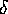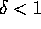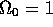Next: Generation of Perturbations Up: Gravitational Instability Previous: Linear Perturbation Theory

### Non-linear Perturbation Theories

Linear perturbation theory gets less accurate the closer the density contrast gets to unity--we are then entering the non-linear regime. Since most of the observed structures in the Universe have density contrasts far in excess of unity, their formation can only be understood by non-linear theory. The boundary between the linear and the non-linear regime is not entirely clear-cut; there is an intermediate regime where fluctuations are ``weakly'' non-linear, meaning thatis close to unity (from above and below). A qualitative description of some of the theories for the weakly non-linear regime will be given in the following.

The density contrast will divide the Universe into several overdense () and underdense () regions. It is reasonable to believe that the overdense regions are the seeds of the structures we see today, and the underdense regions are the observed voids. In the overdense regions, the self-gravity of the local mass concentrations will work against the general expansion; i.e. these regions will expand slower compared to the background universe. Such a region will therefore increase its gravitational potential until it collapses under its own self-gravity. The simplest model is based on the assumption that these overdense regions are spherically symmetric, see Pad and P-80. For a flat universe model (), the perturbations will have a maximum size when[page 280]Pad, which is well inside the non-linear regime. This has been proposed as the origin of spiral and elliptical galaxies, where the type depends of the matter being dissipative or not, respectively. Zel-70a,Zel-70b showed that the first bound systems form as sheet-like mass concentrations, or ``pancakes'', which rules against the spherical symmetry. For example, for an isolated expanding spheroid the expansion along the shorter of the axes will stop quickly Icke,P-80. Thus, spherical symmetry can only be valid when the perturbations are small ().

Numerical N-body simulations have been employed to test the validity of the Zel'dovich approximation works, and they have shown that it works quite well at the beginning of the non-linear stage. Later, however, after the formation of the pancakes, the two methods show differences in the evolution of the density inhomogeneities. For example, the N-body simulations show that the pancakes remain relatively thin, while the Zel'dovich approximation predicts them to thicken. A way to solve this problem, is to introduce a ``viscous'' term to the Zel'dovich approximation, often referred to as the adhesion model Gur. This model gives an analytic solution for irrotational velocity fields, and reproduces the N-body simulations quite well. Although, the main problem with the Zel'dovich approximation is that it breaks down in the presence of singularities.

There have in recent years sprung up other approximations, such as the frozen-flow Mat and frozen-potential Brain. They are both based on the same idea as the Zel'dovich approximation: one chooses a slowly varying physical quantity governed by linear theory and extrapolates it into the non-linear regime. In the Zel'dovich approximation, the velocity of the growth factor is set constant for each particle, while the velocity field of the growth factor is set constant in the frozen-flow approximation and the Newtonian potential is invariable in the frozen-potential approximation. There are also a truncated Zel'dovich approximation, where one removes all the small-scale non-linearities.

A direct method of following the non-linear evolution of the density perturbations is by cosmological N-body simulations (as was used to test the Zel'dovich approximation above). The idea is to describe the matter distribution as a collection of N particles interacting via gravity. The state of the system at any time t is given by the positions and velocities of the particles, which are evolved through a sequence of small time-steps. At each point in time, the force acting on any particle is being determined by considering all the other (N-1) particles, and used to calculate the subsequent state of the system. (More on the N-body algorithms in Section 5.2.) By using a large number of particles in order to imitate a smooth density distribution, one has in the CDM picture been able to, for example, test for different cosmological models and predict the rotational curves of galaxies. Applied to the HDM model, the simulations have brought out several potential problems, which led to a decline in the popularity of that mass model Pad.

The AVP is, as mentioned in Chapter 1, in practice an alternative cosmological N-body method. Both methods considers point-particles in an expanding cosmological model, both methods work best in the quasi-linear regime, and both calculate the motion of the particles using the same equations of motion. The difference lies in the way that these equations of motion are being calculated. This will be clarified later in this thesis.Next: Generation of Perturbations Up: Gravitational Instability Previous: Linear Perturbation Theory

Trond Hjorteland
Mon Jul 5 02:59:28 MET DST 1999